Open Channel Flow

# Open Channel Flow | Mechanical Engineering SSC JE (Technical)

 1 Crore+ students have signed up on EduRev. Have you?

OPEN CHANNEL FLOW

• Flow through open channels is laminar if Reynold’s number is £ 500. If Reynold’s number is > 1000 then flow is called turbulent.

Velocity Distribution

• The contours of equal velocity are called ISOVELS.
• The maximum velocity of the cross section occurs at a distance below the free surface. This dip of the maximum velocity point, giving surface velocities which are less than the maximum velocity, is due to secondary currents and is a function of the aspect ratio (ratio of depth to width) of the channel. Thus, for a deep narrrow channel the location of the maximum velocity point will be much lower from the water surface than for a wider channel of same depth.
• Field observations have shown that the average velocity at any vertical (Vav), occurs at a level of 0.6y0 from the free surface, where yis the depth of flow.
• Further, it is found that,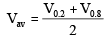where,
V0.2 = velocity at a depth of 0.2 y0 from the free surface.
V0.8 = Velocity at a depth of 0.8 y0 from the free surface.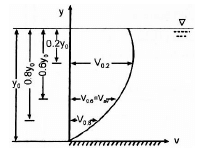Terms related to open channel flow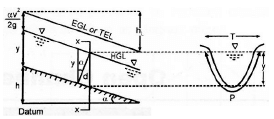(a) Depth of Flow (y) : Vertical distance between the lowest point of the channel section (bed of the channel) to the free liquid surface.
(b) Top width (T) : Width of channel section at free liquid surface.
(c) Wetted Area (A) : Cross sectional area of the channel normal to the direction of flow.
(d) Channel slope (S) : Inclination of channel bed is called channel slope and is given by,

S = tan a » sin a = (h/I)

where h = vertical fall in length ℓ of channel.

(e) Wetted Perimeter and Hydraulic mean depth

• The channel lining (sides and base of the channel) which comes in indirect contact with the liquid stream is called the wetted perimeter (P).
• The Hydraulic mean depth or Hydraulic Radius is the ratio of the wetted area to the wetted perimeter,
(R=A/P)

(f) Hydraulic depth (D) : It is the ratio of wetted area A to the top width T.

(D=A/T)

• It indicates the pressure at various sections along the channel.
• For open channel it coincides with Free liquid surface.

(h) Energy Grade Line (EGL) or Total energy Line (TEL)

• Indicates the total energy of the liquid with respect to a chosen datum.
• The slope of TEL is referred to as hydraulic Slope. It equals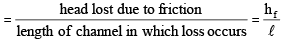Difference between TEL/EGL and HGL at any section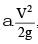where a = Kinetic energy correction factor

Specific Energy

• The total energy of a channel flow referred to a datum is given by :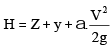If the datum coincides with the channel bed at the section, the resulting expression is known as SPECIFIC ENERGY and is denoted by E. Thus.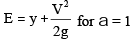• From above equation, if can be noted that while the Total energy always decreases in the downstream, the specific energy always remains constant for a Uniform flow & it can either increases or decrease in varied flow.
• In case of Non uniform flow, if the frictional resistance of flow is neglected, the total energy will be constant at all sections but the specific energy for such flows will be constant only for a horizontal channel, and vary in all other cases.

Critical Depth

• Where Q = constant, the variation of E with y is represented by a cubic parabola, And, there are two positive roots for the equation of E. This indicate that any particular discharge Q1 can be passed in a given channel at two depths and still maintains the same specific energy E. These two possible depths having the same specific energy are known as ALTERNATE DEPTHS.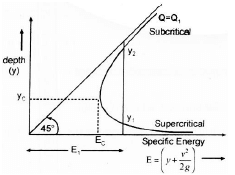• At E = Ec, the two alternate depths merge each other. No value of y can be obtained below Ec. It means, for E < Ec flow is not possible. The condition of Minimum specific energy is known as Critical flow condition and the corresponding depth yc is knwon as Criticial Depth.
• At critical depth, the specific energy is minimum.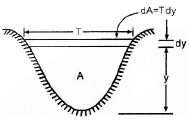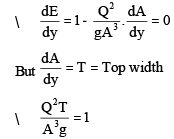Equation (i) & (ii) are basic equations for critical flow conditions in a channel.

• FROUDE NUMBER of the flow is defined as,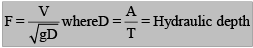at critical flow F = 1 at y = yc

• For alternate depths
y1 < yc : F1 > 1 Þ Super critical flow
y2 > yc : F1 < 1 Þ Sub critical flow.

Uniform Flow

• Possible only in prismatic channels.
• Depth of flow, area of cross section and velocity all remain constant along the channel.
• The total energy line, bottom surface and water surface, all are parallel.

Velocity Formulae in Uniform Flow

(a) Chezy Equation

•  It is given by,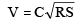where,
V = velocity of flow R = hydraulic radius (A/P)

S = bottom slope

• C is a constant which depends on the nature of the surface and the flow. Dimension of C is [L1/ 2 T - 1 ] It can be made dimensionless by dividing it with √g. C is also known as chezy’s coefficient.

Relationship between ‘C’ and friction factor ‘f’

• For pipe flow, the Darcy - Weisbach equation is :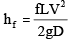where,
hf = head loss due to friction
D = Diameter of pipe
f = friction factor
L = length of pipe

• For circular shapes, hydraulic radius, R, is given by,
(R=D/4)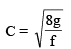2. Manning’s Formula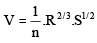where, n = a roughness coefficient known as Manning’s ‘n’.

• Dimension of n is [L- 1/ 3T]

• The manning’s formula is most widely used in uniform flow.

Relationship between Manning’s ‘n’ ‘c’ & ‘f’ :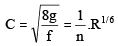(By comparing chezy and Manning’s formula)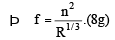(3) Ganguillet and Kutter Formula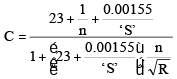where, n = manning’s coefficient.

HYDRAULICALLY-efficient channel sections

• The channel section which conveys maximum discharge for minimum perimeter, with a fixed slope, roughness coefficient and area of flow is called hydraulically efficient section.
• It is also called ‘Best Section’.
(a) Rectangular Section
A = By = Constant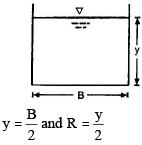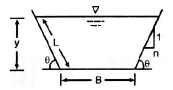A = (B + ny) y = Constant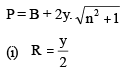(ii) The trapezoidal channel should be part of regular hexagon.

(iii) The side slopes should be 60° with the horizontal.

(iv) Half top width is equal to one of the sloping sides

(c) Triangular Section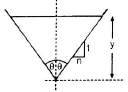(i) q = 45°

(ii)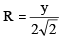(d) Circular Section
Case 1 : Condtion for maximum discharge :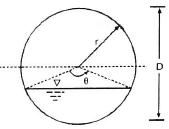y = depth of flow

(i) y » 0.95 D and
(ii) hydraulic radius, R » 0.29 D

Case 2 : Condition for maximum mean velocity

(i)y » 0.81D
(ii)R » 0.30D

Basic Assumptions of Gradually Varied Flow Analysis

(i) The pressure distribution at any section is assumed to be hydrostatic.

(ii) The resistance to flow at any depth is given by the corresponding uniform flow equation, such as Manning’s formula with the condition that the slope term to be used in the equation is energy slope and not the bed slope.

Dynamic Equation of GVF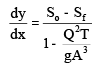Classification of Water Surface Profiles

• It is clear that the gradient of free surface dy/dx may be positive or negative depending on signs of numerator and denominator, which in turn depends on y, yc and yn
• Whether the yn is below or above yc will depend on the classification of be slope.
• Surface profiles are classified by a letter and a number. The letter refers to the bed slope, whcih may be one of the fllowing :

M Mild slope y  n  > yc
C Critical slope yn = yc
S Steep slope yn < yc
H Horizontal So = O

RAPIDLY VARIED FLOW (RVF)

Hydraulic Jump 

• Hydraulic Jump Is an example of steady RVF.
• A hydraulic jump occurs when a super critical stream meets a subcritical stream of sufficient depth. The super critical stream jumps up to meet its alternate depth.
While doing so it generates considerable disturbances in the form of large scale eddies and a reverse flow roller with the result that the jump falls short of its alternate depth. 
• A schematic sketch of jump is as shown below :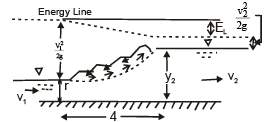
• Section (1), the point of commencement of jump is called TOE of Jump.
• The distance between (1) and (2) is called LENGTH OF JUMP (L J).
• The two depth y1 and y2 at the ends of the jump are called SEQUENT DEPTHS.
• The specific force diagram resembles specific energy diagram.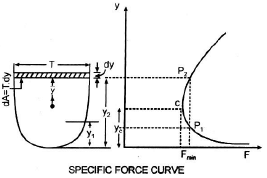From specific force curve we can find the sequent depths y1 and y2 for a given discharge in a given horizontal channel.
Condition for Critical Flow :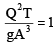Hydraulic Jump in a rectangular channel :

(a) Sequent depth ratio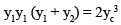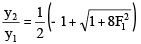F1 = Froude number at (i)

(b) Energy Loss

(i) EL = E1 – E2
(ii)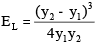(c) Height of Jump = y2 – y

(d) Length of jump = 5 to 7 times Height of Jump

(e) Ratio of energy loss to initial energy :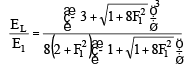Classification of Jumps

 Type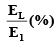F1 Undular Jump                                  1 – 1.7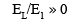Weak Jump                                    1.7 – 2.5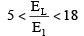Oscillating Jump                            2.5 – 4.5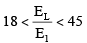Steady Jump                                  4.5 – 9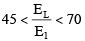Stronger choppy                           > 9.0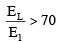Surges

• Surge is an example of unsteady rapidly varied flow.
• It occurs wherever there is a sudden change in the discharge or depth or both.
• Example of such situation is sudden closure of gate.
• A surge producing increase in depth is called Positive surge.
• A surge which causes a decrease in depth is known as Negative surge.
• Positive surge is STABLE and having steep fronts, but, negative surges are UNSTABLE (Their form changes with advance of the surge).
The document Open Channel Flow | Mechanical Engineering SSC JE (Technical) is a part of the Mechanical Engineering Course Mechanical Engineering SSC JE (Technical).
All you need of Mechanical Engineering at this link: Mechanical Engineering

## Mechanical Engineering SSC JE (Technical)

6 videos|97 docs|55 tests

## Mechanical Engineering SSC JE (Technical)

6 videos|97 docs|55 tests

Track your progress, build streaks, highlight & save important lessons and more!

,

,

,

,

,

,

,

,

,

,

,

,

,

,

,

,

,

,

,

,

,

;